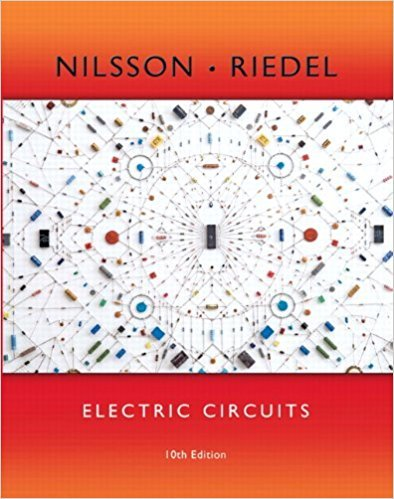×
×

# Solved: Use the principle of superposition to find the voltage in the circuit in FigISBN: 9780133760033 390

## Solution for problem 4.96 Chapter 4

Electric Circuits | 10th Edition

• Textbook Solutions
• 2901 Step-by-step solutions solved by professors and subject experts
• Get 24/7 help from StudySoup virtual teaching assistantsElectric Circuits | 10th Edition

4 5 1 398 Reviews
24
2
Problem 4.96

Use the principle of superposition to find the voltage in the circuit in Fig. P4.96.

Step-by-Step Solution:
Step 1 of 3

MODULE 4 ARTICLE I. INFERENCE FOR POPULATION MEANS 1) Confidence Intervals a. We can find confidence intervals of two differencesample means 2) Known Population Variances a. Let X and Y be to independent binomial random variables. b. A 100(1-α)% CI for the difference of the two meansfrom each random...

Step 2 of 3

Step 3 of 3

##### ISBN: 9780133760033

The answer to “Use the principle of superposition to find the voltage in the circuit in Fig. P4.96.” is broken down into a number of easy to follow steps, and 15 words. This full solution covers the following key subjects: . This expansive textbook survival guide covers 107 chapters, and 1357 solutions. This textbook survival guide was created for the textbook: Electric Circuits, edition: 10. The full step-by-step solution to problem: 4.96 from chapter: 4 was answered by , our top Engineering and Tech solution expert on 03/13/18, 07:48PM. Electric Circuits was written by and is associated to the ISBN: 9780133760033. Since the solution to 4.96 from 4 chapter was answered, more than 215 students have viewed the full step-by-step answer.

Unlock Textbook Solution